Function Repository Resource:

Determine whether an expression represents a quadratic function of a given set of variables

Contributed by: Paco Jain (Wolfram Research)
 ResourceFunction["QuadraticFunctionQ"][expr,var] returns True if expr is a quadratic function of the symbol var, and returns otherwise. ResourceFunction["QuadraticFunctionQ"][expr,{var1,var2, …}] returns True if expr is a quadratic function of each of the symbols vari, considered together, and returns False otherwise.

## Details and Options

If expr is of the form lhs==rhs, ResourceFunction["QuadraticFunctionQ"][expr, ] is equivalent to ResourceFunction["QuadraticFunctionQ"][lhs - rhs, ]
An expression is determined to be quadratic if and only if it is a PolynomialQ in the variable(s) of the second argument, has at least one term of cumulative order 2 and has no terms of cumulative order greater than 2.

## Examples

### Basic Examples (2)

Test whether an expression represents a quadratic function of a given variable:

 In:=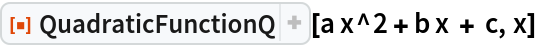Out=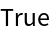In:=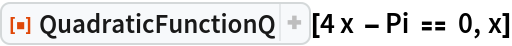Out=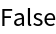Test whether a given expression represents a quadratic function of a list of variables:

 In:=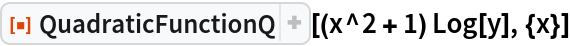Out=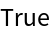In:=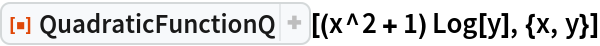Out=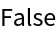In:=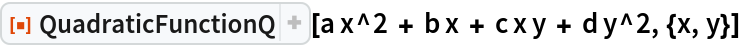Out=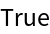### Properties and Relations (3)

Variables listed in the second argument are considered together:

 In:=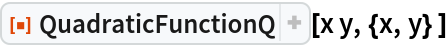Out=In:=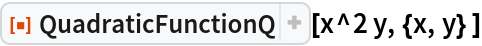Out=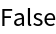If high-order terms in expr do not explicitly vanish, QuadraticFunctionQ[expr, ] returns False:

 In:=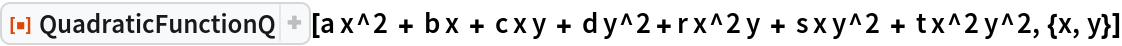Out=Explicitly taking the cubic and quartic coefficients of the previous input to be zero yields True:

 In:=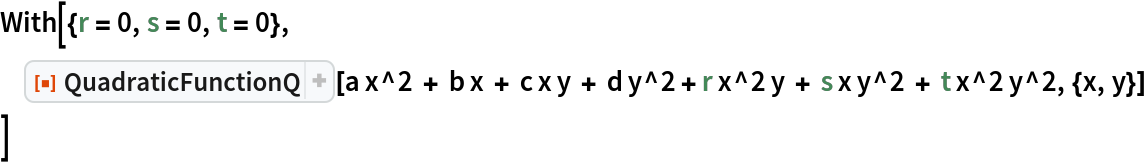Out=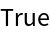Expressions that lack an explicit quadratic term are not considered to be QuadraticFunctionQ:

 In:=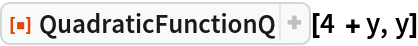Out=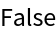## Requirements

Wolfram Language 11.3 (March 2018) or above

## Version History

• 1.0.0 – 20 March 2019